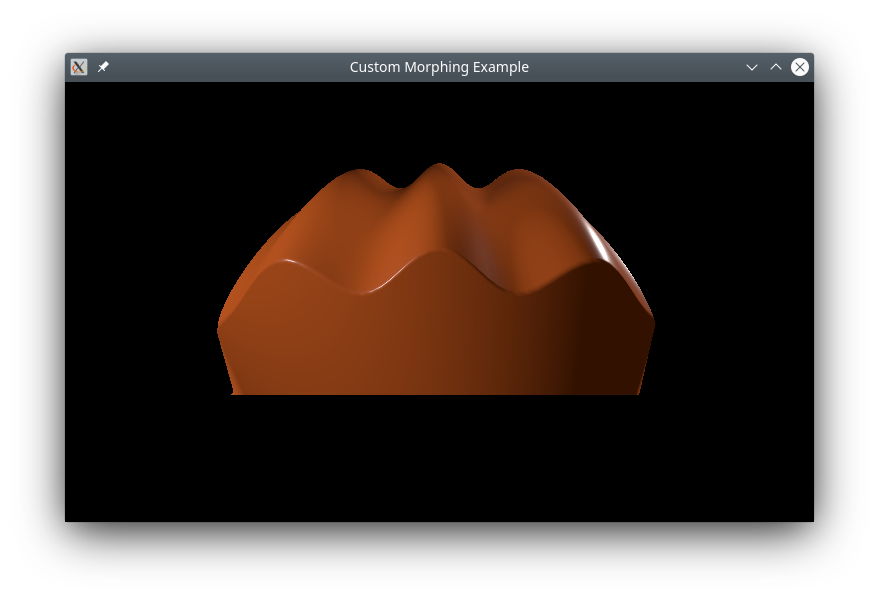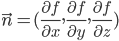# Qt Quick 3D - Custom Morphing Animation

Demonstrates writing C++ custom geometry with a morph target.This example shows how to define a complex custom geometry in C++ that contains a base shape and a morph target, with normal vectors for both.

#### Custom geometry

The main part of this example is creating a custom geometry with a morph target. We do this by subclassing QQuick3DGeometry:

```class MorphGeometry : public QQuick3DGeometry
{
Q_OBJECT
QML_NAMED_ELEMENT(MorphGeometry)
Q_PROPERTY(int gridSize READ gridSize WRITE setGridSize NOTIFY gridSizeChanged)

public:
MorphGeometry(QQuick3DObject *parent = nullptr);

int gridSize() { return m_gridSize; }
void setGridSize(int gridSize);

signals:
void gridSizeChanged();

private:
void calculateGeometry();
void updateData();

QList<QVector3D> m_positions;
QList<QVector3D> m_normals;

QList<QVector3D> m_targetPositions;
QList<QVector3D> m_targetNormals;

QList<quint32> m_indexes;

QByteArray m_vertexBuffer;
QByteArray m_indexBuffer;

int m_gridSize = 50;
QVector3D boundsMin;
QVector3D boundsMax;
};```

The constructor defines the layout of the mesh data:

```MorphGeometry::MorphGeometry(QQuick3DObject *parent)
: QQuick3DGeometry(parent)
{
updateData();
}```

The function `updateData` performs the actual uploading of the mesh geometry:

```void MorphGeometry::updateData()
{
clear();
calculateGeometry();

QQuick3DGeometry::Attribute::ComponentType::F32Type);
QQuick3DGeometry::Attribute::ComponentType::F32Type);

QQuick3DGeometry::Attribute::ComponentType::F32Type);
QQuick3DGeometry::Attribute::ComponentType::F32Type);

QQuick3DGeometry::Attribute::ComponentType::U32Type);

const int numVertexes = m_positions.size();
m_vertexBuffer.resize(numVertexes * sizeof(Vertex));
Vertex *vert = reinterpret_cast<Vertex *>(m_vertexBuffer.data());

for (int i = 0; i < numVertexes; ++i) {
Vertex &v = vert[i];
v.position = m_positions[i];
v.normal = m_normals[i];
v.targetPosition = m_targetPositions[i];
v.targetNormal = m_targetNormals[i];
}

setStride(sizeof(Vertex));
setVertexData(m_vertexBuffer);
setPrimitiveType(QQuick3DGeometry::PrimitiveType::Triangles);
setBounds(boundsMin, boundsMax);

m_indexBuffer = QByteArray(reinterpret_cast<char *>(m_indexes.data()), m_indexes.size() * sizeof(quint32));
setIndexData(m_indexBuffer);
}```

We call `updateData` from the constructor, and when a property has changed.

The function `calculateGeometry` contains all the tedious mathematics to calculate the shapes and normal vectors. It is specific to this example, and the code will not be explained in detail. In general: to implement smooth shading, it's necessary to calculate the normal vector for each vertex. Mathematically, the normal vector can be calculated from the partial derivatives of the function describing the plane:In this example, we make it simple by using a cosine wave for the base shape, knowing that its derivative is a sine function.

In practice, normal vectors can often be determined by geometric reasoning. For the morph target, we use the fact that any vector from the center of a sphere to the surface will be normal to the sphere at that point. Note that normal vectors in QtQuick3D must have unit length, which can be done by using QVector3D::normalized().

#### QML part

We define a morph target that corresponds to the one we created in the custom geometry, and do an animation on the weight, so it will cycle between the two shapes:

```MorphTarget {
id: morphtarget
attributes: MorphTarget.Position | MorphTarget.Normal
SequentialAnimation on weight {
PauseAnimation { duration: 1000 }
NumberAnimation { from: 0; to: 1; duration: 4000 }
PauseAnimation { duration: 1000 }
NumberAnimation { from: 1; to: 0; duration: 4000 }
loops: Animation.Infinite
}
}```

Finally, we create a model using our custom geometry, and apply the morph target to it:

```Model {
y: -1
geometry: MorphGeometry {}
morphTargets: [ morphtarget ]
materials: material
}```

Files: